### Home > CALC > Chapter Ch3 > Lesson 3.3.1 > Problem3-88

3-88.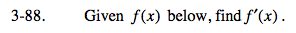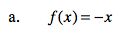You should know by now that a derivative is a slope function. Notice that this is a linear equation, and linear equations have constant slopes.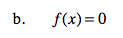SImilarly, this is a horizontal line. What is the slope of a horizontal line? That will also be the derivative.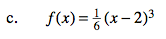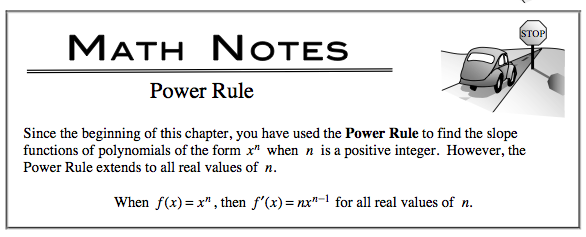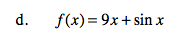Recall that the derivative of sin(x) is cos(x).